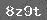# 8的乘法口诀及求商习题精选

1．判断题

2×8和8×2表示的意义是一样的． ( )

2．在○里填上+、-、×、÷符号．

4○8=32 2○8=16

3．把口诀填完整, 并写出两个乘法算式．

( )八四十   1.__________2.__________

4. 把口诀补充完整, 再写两个乘法算式．

(1)三八( ) 六八( )

____________ __________

____________ __________

(2)五八( ) 七八( )

____________ __________

____________ __________

(3)四八( ) 二八( )

____________ __________

____________ __________

5. ( )×6=48 8×( )=40 ( )×8=48

8×( )=56 ( )×8=16 7×( )=28

( )×8=32 3×( )=24 ( )×( )=8

1. 填口诀。

( )( )三十二　　( )八二十四
六( )四十八　　　二八( )

2. 填空。

9+9=6×( ) 32÷8=( ) ÷2
3×4= ×2 ×8 < 7×3

3. 在正确的答案下面打勾

①有2个盘，一个盘里放8个苹果，一共有几个苹果?
A.8×2　　B.2+8　　C.8÷2
②有2个盘，一个盘里放8个苹果，另一个盘里放4个苹果，一共有几个苹果?
A.8×2　　B.4×2　　C.8+4
③第二小组做的好事是第一小组的3倍，第一小组做了6件，第二小组做了几件?
A.3+6　　B.6÷3　　C.6×3

4．①补充条件后，使之能用减法计算。
②填上第二个条件后，使之计算结果是24只黑兔。
③如果第一个条件不变，第二个条件是2只黑兔，问题可以怎么变?

5．

①3连续加3，得出24需要加几次?
②48连续减6，得出0需要减几次?
③80连续减8，得出24需要减几次?

1、填口诀

（    ）×（    ）=8         …

（   ）÷（   ）=8        …

2、下面各数中，哪两个数相除的商是8？（写出算式）

2    5   48   7   40   6   56   3    16    24

3、下面哪两个数有倍数关系？写出算式。（可重复使用）

20      16      24      4      8

4、在□里填上数

3 ×□ + 2 = 20            24 ÷□ = 12 ÷ 4

2 ×□ = 4 × 4            40 - 8 =  8 × □

（9 - □）×  6 = 24        □ ÷ 8 > 42 ÷ 6

5、用4、32、8三个数编一道乘法，两道除法应用题。

6、### 相关推荐

 留言与评论（共有 0 条评论）

 验证码：